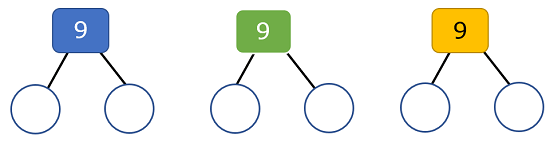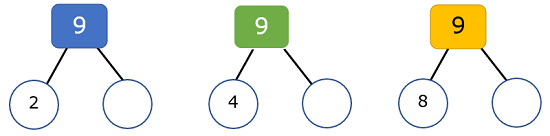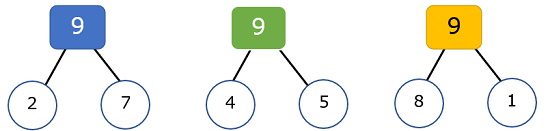## Number Bonds

#### Grade 1 Operations - OA.6

A number bond is pairing values together where the sum is equal to a given number. A good example would be the number bond for the number 8: 0 + 8, 1 + 7, 2 + 6, 3 + 5, 4 + 4
Due the commutative property of addition, the same sums with addends flipped would be included too. While this concept can seem pretty simple, it is super helpful to help students learn about structure and consistency with their lower math facts. This is a nice method that does not require students to boldly just memorize their facts. It is really helpful to do this as a class and ask them, "How many math facts can you think of for the number bond of _____?" I find it especially helpful to have them write a single math fact for each horizontal line. This also them to form a mental picture of the fact much quicker and in a more methodical manner. Below you will find our lessons and worksheets that can help make number bonds much more approachable for your students. We will start out with considerably basic math facts and as you move on to the homework and practice sheets below, we will advance to double digit values and sums.

• Step-by-Step Lesson- You will see visually how to use this skill and how it applies to math facts.

• Guided Lesson - We stick with a very simple approach to get you started with this skill.

• Detailed Guided Lesson Explanation - This walks through all the steps that are required to complete these problems.

• Independent Practice Worksheet - This is you first chance to get after it with this skill across eight problems. It is well spaced out.

• Matching Worksheet - You will match the missing value to each number bond set up.
•#### Homework Sheets

As you progress through these assignments you will notice that they get progressively more difficult. That is by design to help elevate your skills.

• Homework 1 - This is spider worksheets, as we call it. There is a central sum that each of the arms must match.
• Homework 2 - We actually apply this skill to basic single step algebra problems.
• Homework 3 - For each problem you will have a bar model that is incomplete. Write the value in the missing bar.

#### Practice Worksheets

It is a good idea to review your basic addition and subtraction facts along with these problems to make it more automatic for students.

• Practice 1- There are some double digit answers in here, it is a step in the right direction.
• Practice 2- We varied the alignment of the addends and missing addends here to keep students on their toes.
• Practice 3 - This adds a nice visual to help reinforce the values that are present. All of these values are a multiple of ten based out of a hundred, so the answer will be as well.

#### Quizzes

We saved the best for last here. From colors to creative uses of ranges, we pulled out all the stops here.

• Quiz 1- We work off ten, which makes this a nice simple quiz for you.
• Quiz 2- The values of both the sums and addends are larger than previous versions.
• Quiz 3 - This is the first time the bar models we not round numbers. It is still based off of a hundred though.

### How Do You Use Number Bonds?

Take a look at how this work. Below you will see a framework for a number bond.Step 1) In a number bond the number on the top of the bond diagram represents the value that you would get when you add the two values below it (in the circles). Our first step is therefore to choose a number that would be in one of the circles for each bond. That value can be any number equal to or less than the value of the bond. So, we will just choose 3 numbers to start with. In this case, we will choose 2, 4, 8. We will place these values in one of the circles for each number bond.Step 2) Complete the math facts that make the sum of the number bond number. If we were to restate these values, in order, they would be 2 + __ = 9, 4 + __ = 9, 8 + __ = 9. We will then complete the math facts below.### Why Is This Skill Important?

While at first glance this may just seem like a fun way to review basic math facts or a pointless math puzzle of sorts, this skill really helps students begin to understand the language of math. Students that have a solid grasp of the concepts that we explore here will have the ability to compose and decompose values with greater ease. Students will also be able to form a mental picture that envisions these pieces fitting into a whole. Yes, this skill does help students reinforce and eventually master their fact families. Students will begin to see how addition and subtraction boldly complement one another. As we can see by any of the problems, we are also establishing our groundwork for working with algebra and the equation that so often pop up in that environment.